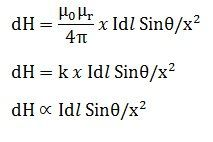# Biot Savart Law

The Biot Savart Law is used to determine the magnetic field intensity H near a current-carrying conductor or we can say, it gives the relation between magnetic field intensity generated by its source current element. The law was stated in the year 1820 by Jean Baptisle Biot and Felix Savart. The direction of the magnetic field follows the right hand rule for the straight wire. Biot Savart law is also known as Laplace’s law or Ampere’s law.

Consider a wire carrying an electric current I and also consider an infinitely small length of a wire dl at a distance x from point A.

The magnetic intensity dH at a point A due to current I flowing through a small element dl is

1. Directly proportional to current (I)
2. Directly proportional to the length of the element (dl)
3. Directly proportional to the sine of angle θ between the direction of current and the line joining the element dl from point A.
4. Inversely proportional to the square of the distance (x) of point A from the element dl.where k is constant and depends on the magnetic properties of the medium.µ0 = absolute permeability of air or vacuum and its value is 4 x 10-7 Wb/A-m
µr= relative permeability of the medium.

### 2 thoughts on “Biot Savart Law”

1. magnetic field strength (H) does not depend on permiability of material. hence permiability term will not come into picture while writing the formula.

am i right?

2. Circuit globe explains Biot Savart law from elementary to advance level. An individual researcher like me can benefit a lot reading such tutorials.